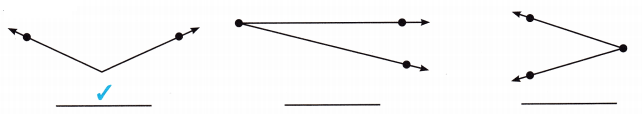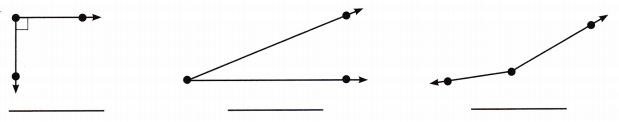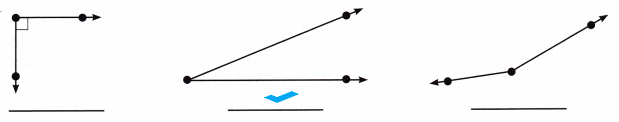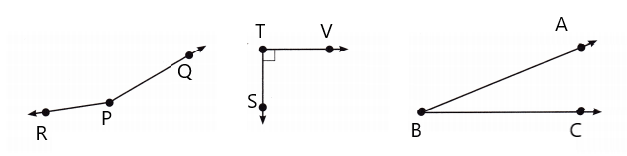# McGraw Hill Math Grade 4 Chapter 12 Lesson 3 Answer Key Acute, Right, and Obtuse Angles

Practice the questions of McGraw Hill Math Grade 4 Answer Key PDF Chapter 12 Lesson 3 Acute, Right, and Obtuse Angles to secure good marks & knowledge in the exams.

## McGraw-Hill Math Grade 4 Answer Key Chapter 12 Lesson 3 Acute, Right, and Obtuse Angles

Solve

Question 1.
Which figure is an obtuse angle? Place a check mark under the correct answer.Explanation:
The first figure is an obtuse angle
So, i placed a check mark under the first figure.

Question 2.
Which figure is an acute angle? Place a check mark under the correct answer.Explanation:
The second figure is an acute angle
So, i placed a check mark under the second figure.

Question 3.
Draw an obtuse angle. Label it ∠RPQ. Draw a right angle. Label it ∠STV. Draw an acute angle. Label it ∠ABC.